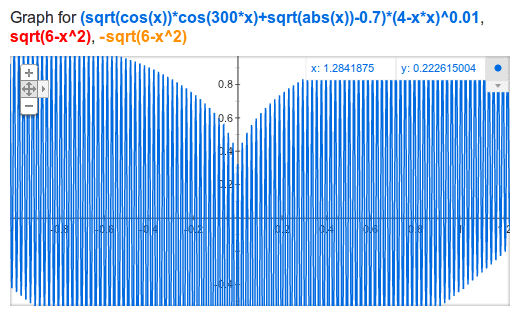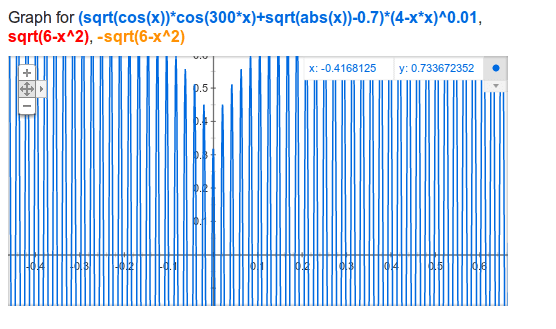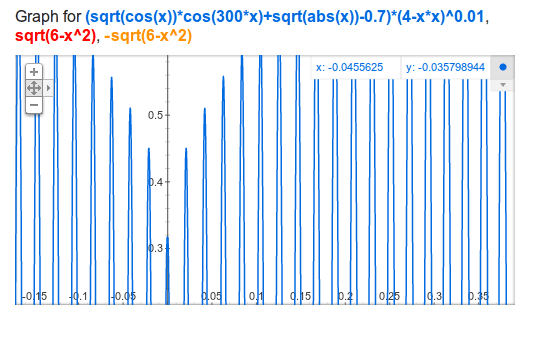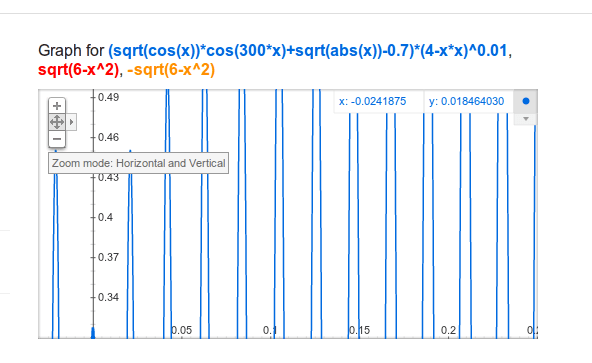Coffee Room
Discuss anything here - everything that you wish to discuss with fellow engineers.
12915 Members
Join this group to post and comment.Kaustubh Katdare • Feb 9, 2012

# Google Valentine Day Easter Egg: Algebraic Equation!

If you want to impress your friends with your Google search abilities; enter the following search query in Google search bar and hit enter.

`sqrt(cos(x))*cos(300x)+sqrt(abs(x))-0.7)*(4-x*x)^0.01, sqrt(6-x^2), -sqrt(6-x^2) from -4.5 to 4.5`
☕ What gives?Valentine Day does not exist for year 2012!

Want proof?

Here: 14-02-12 = 0

😁Anoop Kumar • Feb 10, 2012
This one looks better:
Copy n paste in google

```
sqrt(cos(x))*cos(180x)+sqrt(abs(x))-0.7)*(4-x*x)^0.01, sqrt(6-x^2), -sqrt(6-x^2) from -4 to 4

```
hit enterAnoop Kumar • Feb 10, 2012
The_Big_K
If you want to impress your friends with your Google search abilities; enter the following search query in Google search bar and hit enter.

`sqrt(cos(x))*cos(300x)+sqrt(abs(x))-0.7)*(4-x*x)^0.01, sqrt(6-x^2), -sqrt(6-x^2) from -4.5 to 4.5`
☕ What gives?
There is warning in your code: The function might not be plotted correctly. 😛😁Kaustubh Katdare • Feb 10, 2012
ianoop
There is warning in your code: The function might not be plotted correctly. 😛😁
Looks like you didn't copy the complete code (there's a horizontal scrollbar). Or maybe Google doesn't heart you? 😒

Kidding!ianoop
This one looks better:
Copy n paste in google

```
sqrt(cos(x))*cos(180x)+sqrt(abs(x))-0.7)*(4-x*x)^0.01, sqrt(6-x^2), -sqrt(6-x^2) from -4 to 4

```
hit enter
This is kinda corrugated! Biggie's one was the BEST! 😛circularsquare • Feb 10, 2012
When I saw it at first I wracked my brains over how the plot is 'shaded'. Because by definition a function is such that for a particular value of x , there is only one value of y.

But I noticed that the image is a very zoomed out version of the plot. If you zoom in it has got closely spaced sine-like curve. The zoomed-out version of the plot & the closely-spaced zero crossings give an impression of 'shaded' region. So it is indeed a function with each value of x having at most one value of y.

Nice job.